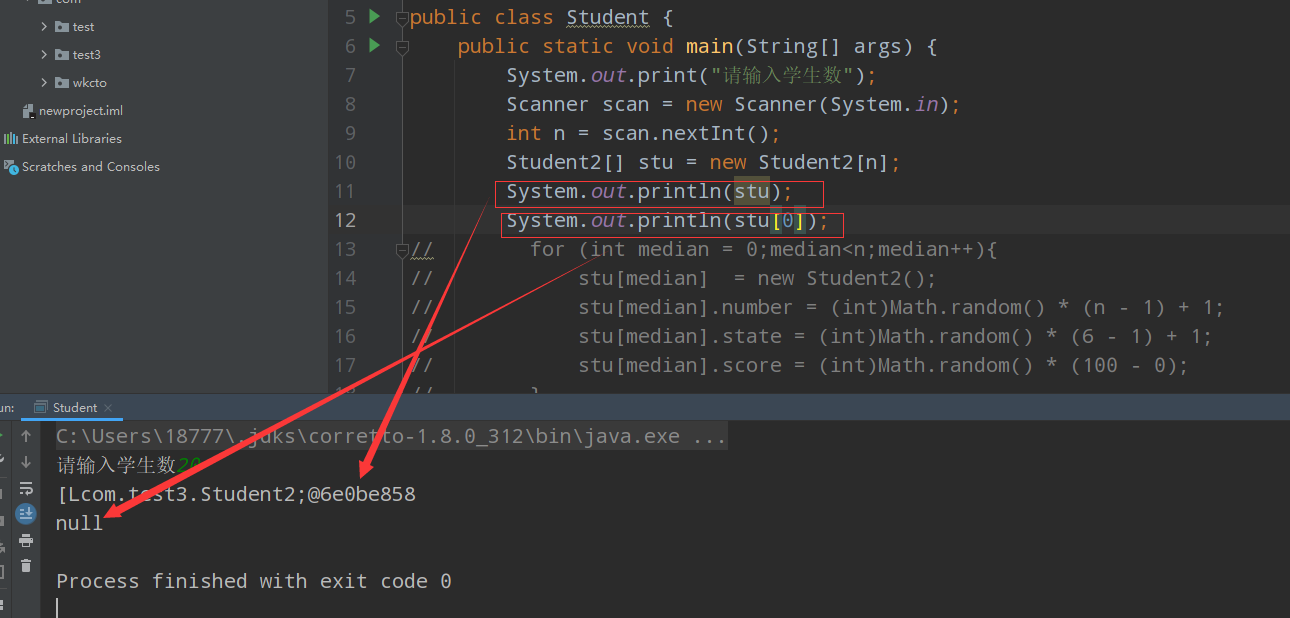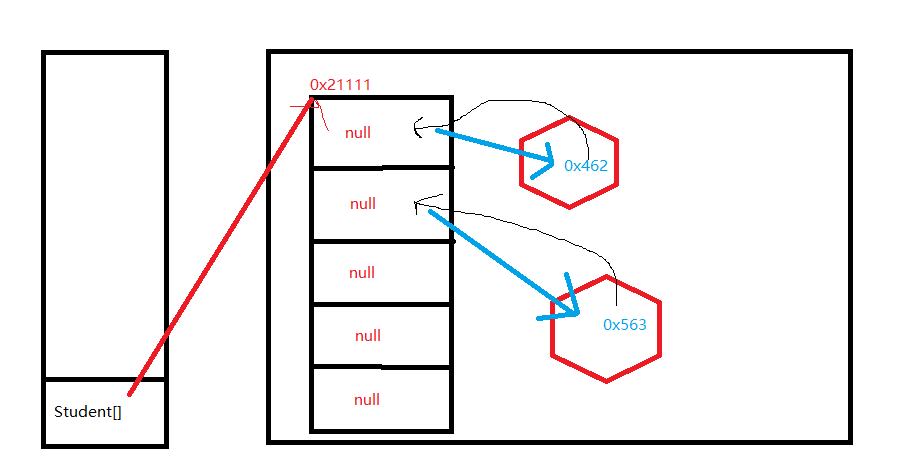# java语言-对象数组的内存解析

## 遇到的问题代码及解决

• 创建一个类
``````class Student2{

int number;
int state;
int score;
}
``````

• 创建对象数组
``````Student2[] stu = new Student2[n];
``````• 给数组中的每一个对象数组赋值
``````for (int median = 0;median<n;median++){

stu[median]  = new Student2();
//利用random随机赋值
stu[median].number = (int)Math.random() * (n - 1) + 1;
stu[median].state = (int)Math.random() * (6 - 1) + 1;
stu[median].score = (int)Math.random() * (100 - 0);
}
```````````` stu[median]  = new Student2();
``````

``````class Information{

int number;
int state;
int score;
}
``````

``````  Information infor = new Information();
``````

• 赋值操作
``````            stu[median].number = (int)Math.random() * (n - 1) + 1;
stu[median].state = (int)Math.random() * (6 - 1) + 1;
stu[median].score = (int)Math.random() * (100 - 0);
``````

• 浏览(39)
• 浏览(29)
• 浏览(26)
• 浏览(24)
• 浏览(22)
• 浏览(22)
• 浏览(22)
• 浏览(22)
• 浏览(21)
• 浏览(18)